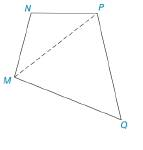Chapter 2.4, Problem 42E### Elementary Geometry for College St...

6th Edition
Daniel C. Alexander + 1 other
ISBN: 9781285195698

#### Solutions

Chapter
Section### Elementary Geometry for College St...

6th Edition
Daniel C. Alexander + 1 other
ISBN: 9781285195698
Textbook Problem
1 views

# A polygon with four sides is called quadrilateral. Consider the figure and the dashed auxiliary line. What is the sum of the measures of the four interior angles of this (or any other) quadrilateral?To determine

The sum of the measures of the four interior angles of the quadrilateral.

Explanation

Given:

Figure (1)

Properties:

The sum of the three angles in a triangle is 180°.

Calculation:

In triangle, ΔMNP

mNPM+mNMP+mN=180°(1)

In triangle, ΔMPQ

mMPQ+mPMQ+mQ=180°(2)

From the figure (1) the angle mNMQ and the mNPQ can be written as,

mNMQ=mNMP+mPMQ

mNPQ=mNPM+mMPQ

Calculate the sum of the measures of the four interior angles of the quadrilateral.

x=mNMQ+mQ+mNPQ+mN(3)

Here, the measures of the four interior angles of the quadrilateral is x

### Still sussing out bartleby?

Check out a sample textbook solution.

See a sample solution

#### The Solution to Your Study Problems

Bartleby provides explanations to thousands of textbook problems written by our experts, many with advanced degrees!

Get Started

#### Sketch the graphs of the equations in Exercises 512. xy=2x3+1

Finite Mathematics and Applied Calculus (MindTap Course List)

#### Simplify: 4854

Elementary Technical Mathematics

#### Multiply. (1+x)2

Trigonometry (MindTap Course List)

#### What graph has f′(2) > 0 and f″(2) < 0?

Study Guide for Stewart's Single Variable Calculus: Early Transcendentals, 8th